### C++ using 编译指令与名称冲突

`using` 编译指令：它由名称空间名和它前面的关键字 `using namespace` 组成，它使名称空间中的所有名称都可用，而不需要使用作用域解析运算符。在全局声明区域中使用 `using` 编译指令，将使该名称空间的名称全局可用；在函数或代码块中使用 `using` 编译指令，将使其中的名称在该函数或代码块中可用。当包含 `using` 声明的最小声明区域中已经声明了和名称空间中相同的名称时，若仍使用 `using` 声明导入该名称空间的同名名称，则这两个名称将会发生冲突，编译器会报错。`using` 声明不同的是，`using` 编译指令会进行名称解析，在一些时候名称空间的变量会被同区域声明的同名变量隐藏，不会出现名称冲突的报错。但在另一些情况下，使用 `using` 编译指令仍会出现名称冲突的报错，下面对此进行总结，测试所用的环境为 Microsoft Visual Studio 2019 以及 QT 5.9.2 MinGW 32bit

## 1 using 编译指令与同名全局变量

### 1.1 在同名全局变量声明前使用

``````#include <iostream>

//自定义名称空间
namespace Jack {
double pail = 1;
}

//在同名全局变量声明前使用
using namespace Jack;

//在全局名称空间中定义变量
double pail = 2;

//测试
int main()
{
using namespace std;

//使用
cout << pail << endl;
cout << ::pail << endl;
cout << Jack::pail << endl;

return 0;
}
``````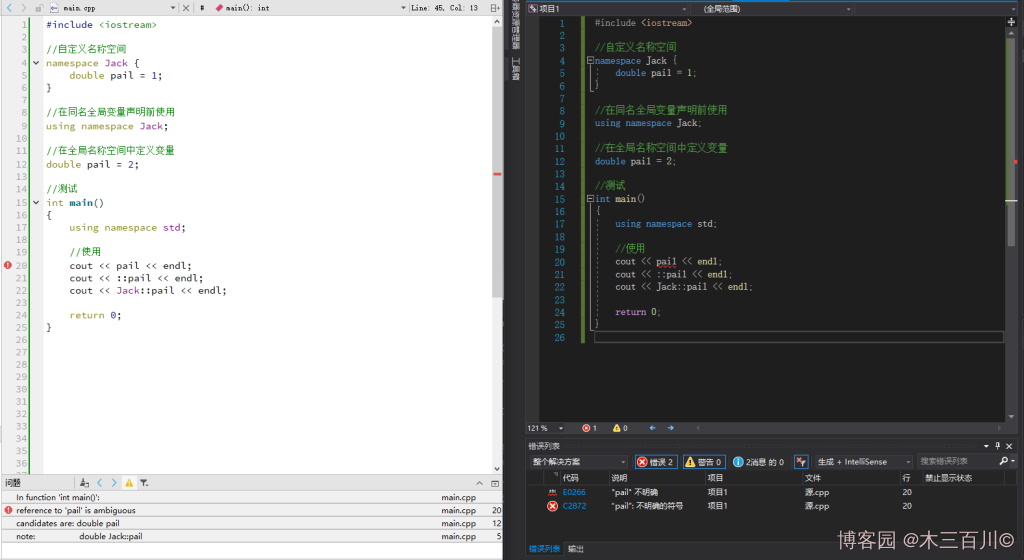### 1.2 在同名全局变量声明后的全局区中使用

``````#include <iostream>

//自定义名称空间
namespace Jack {
double pail = 1;
}

//在全局名称空间中定义变量
double pail = 2;

//在同名全局变量声明后使用
using namespace Jack;

//测试
int main()
{
using namespace std;

//使用
cout << pail << endl;
cout << ::pail << endl;
cout << Jack::pail << endl;

return 0;
}
``````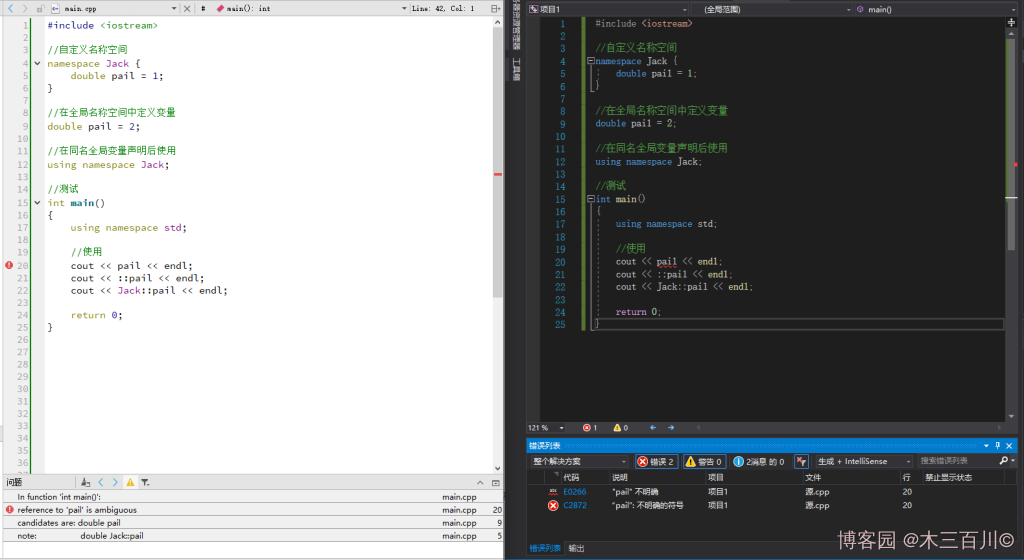### 1.3 在同名全局变量声明后的代码块中使用

``````#include <iostream>

//自定义名称空间
namespace Jack {
double pail = 1;
}

//同名全局变量声明
double pail = 2;

//测试
int main()
{
using namespace std;

//使用
using namespace Jack;
cout << pail << endl;
cout << ::pail << endl;
cout << Jack::pail << endl;

return 0;
}
``````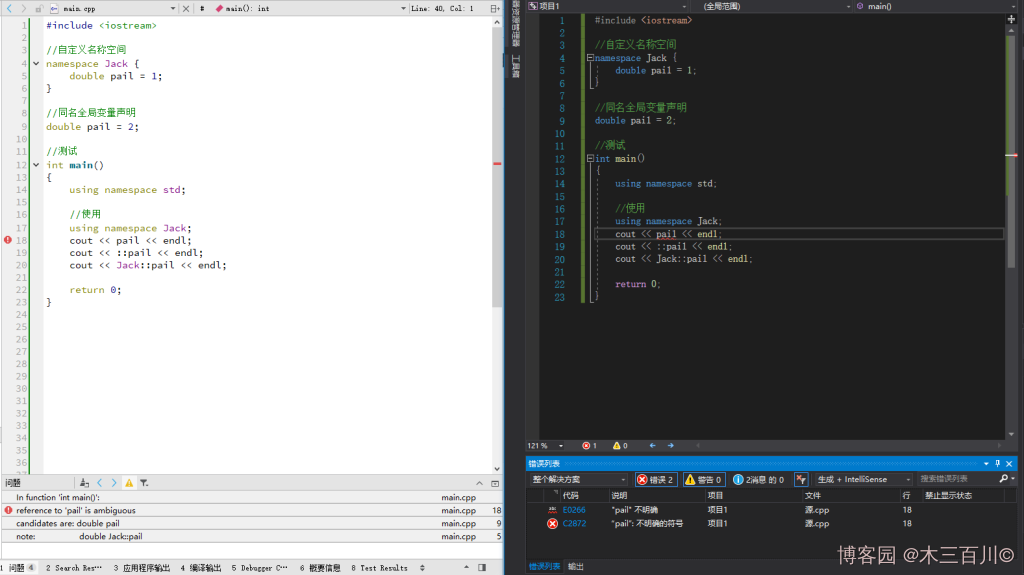## 2 using 编译指令与同名局部变量

### 2.1 在同名局部变量声明前的全局区中使用

``````#include <iostream>

//自定义名称空间
namespace Jack {
double pail = 1;
}

//在同名局部变量声明前的全局区中使用
using namespace Jack;

//测试
int main()
{
using namespace std;

//同名局部变量
double pail = 2;

//使用
cout << pail << endl;       //结果为2
cout << ::pail << endl;     //结果为1
cout << Jack::pail << endl; //结果为1

return 0;
}
``````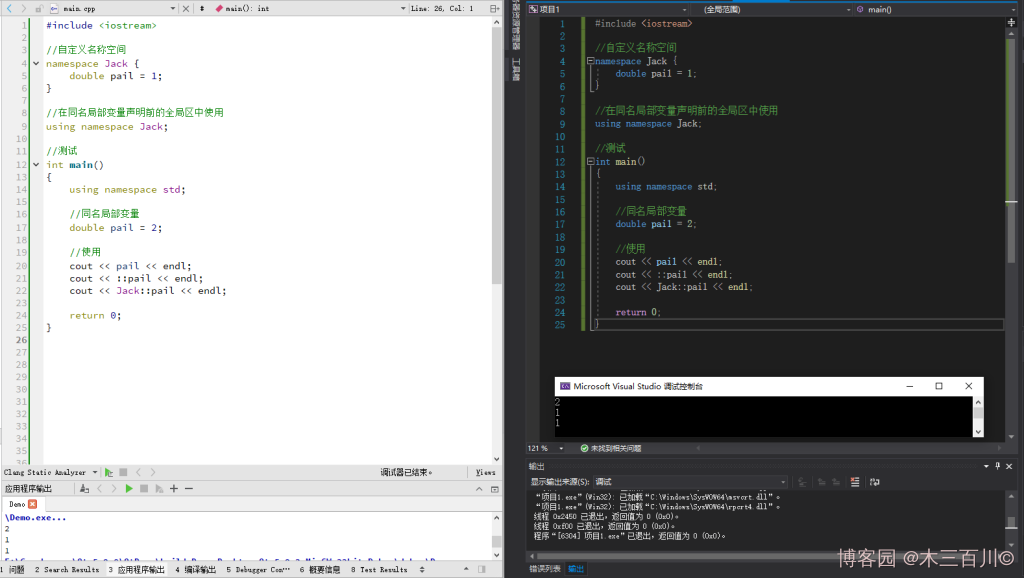### 2.2 在同名局部变量声明前的代码块中使用

``````#include <iostream>

//自定义名称空间
namespace Jack {
double pail = 1;
}

//测试
int main()
{
using namespace std;

//在同名局部变量声明前的代码块中使用
using namespace Jack;

//同名局部变量
double pail = 2;

//使用
cout << pail << endl;       //结果为2
cout << Jack::pail << endl; //结果为1

return 0;
}
``````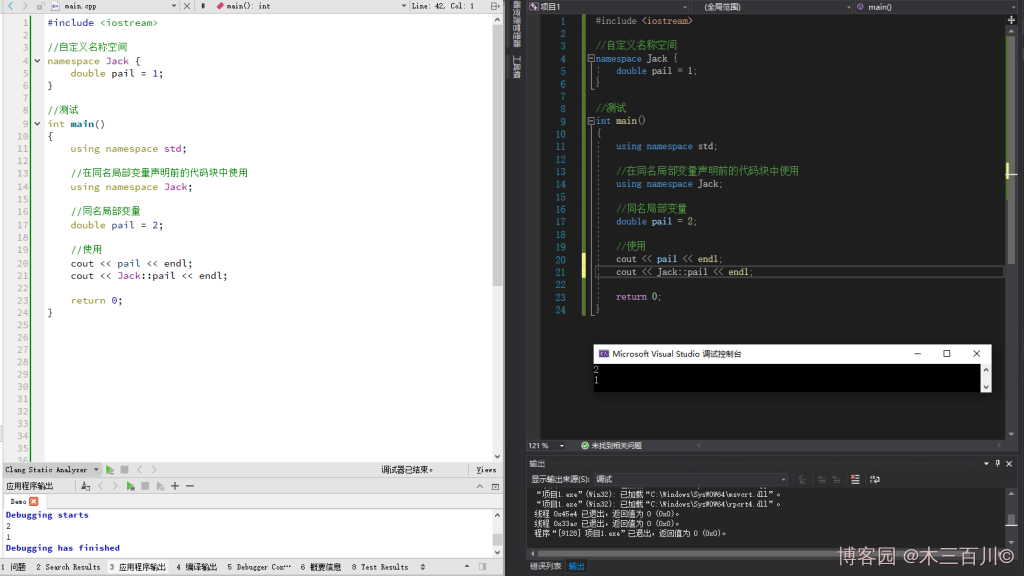### 2.3 在同名局部变量声明后使用

``````#include <iostream>

//自定义名称空间
namespace Jack {
double pail = 1;
}

//测试
int main()
{
using namespace std;

//同名局部变量
double pail = 2;

//在同名局部变量声明后的代码块中使用
using namespace Jack;

//使用
cout << pail << endl;       //结果为2
cout << Jack::pail << endl; //结果为1

return 0;
}
``````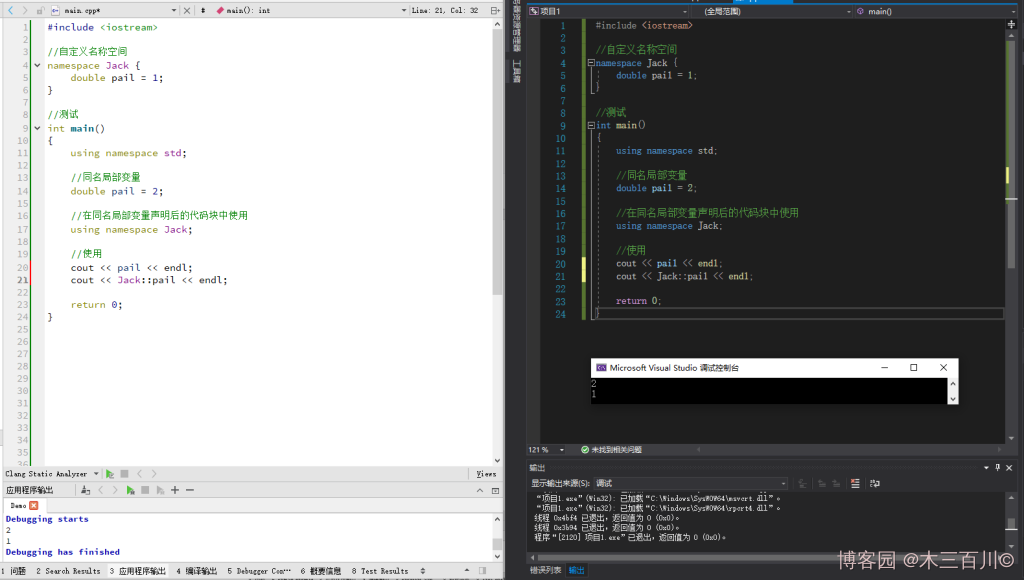## 3 不同名称空间中的同名变量

### 3.1 using 编译指令位置都在全局区中

``````#include <iostream>

//自定义名称空间
namespace Jack {
double pail = 1;
}
namespace Rose {
double pail = 2;
}

//都在全局区中
using namespace Jack;
using namespace Rose;

//测试
int main()
{
using namespace std;

//使用
cout << pail << endl;

return 0;
}
``````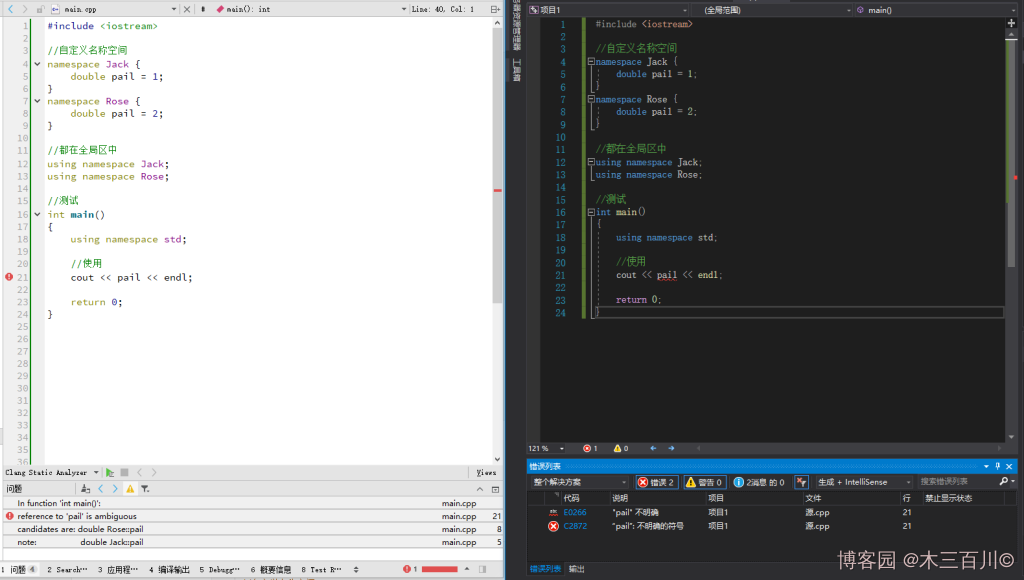### 3.2 using 编译指令位置都在代码块中

``````#include <iostream>

//自定义名称空间
namespace Jack {
double pail = 1;
}
namespace Rose {
double pail = 2;
}

//测试
int main()
{
using namespace std;

//都在代码块中
using namespace Jack;
using namespace Rose;

//使用
cout << pail << endl;

return 0;
}
``````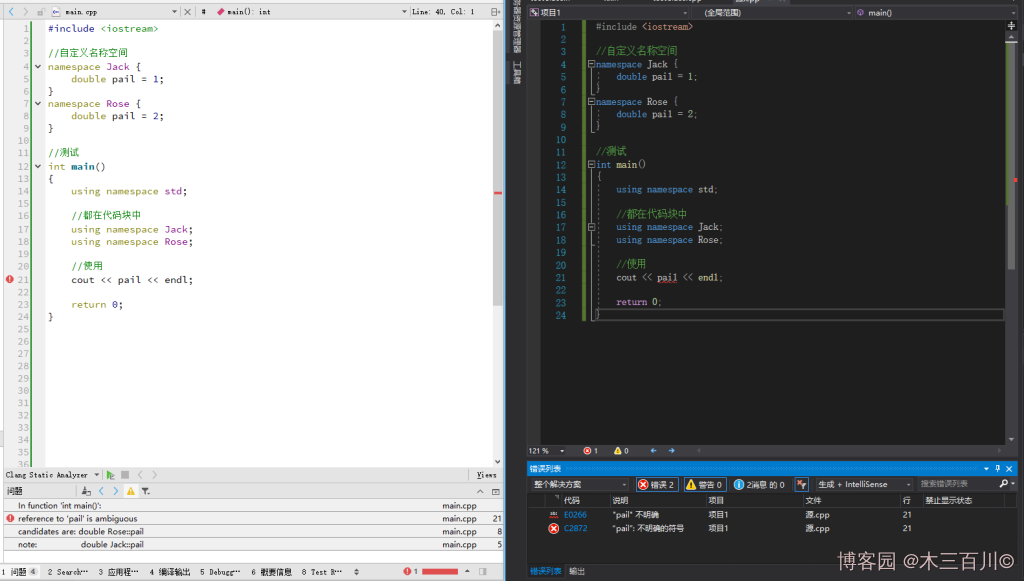### 3.3 using 编译指令位置不同区

``````#include <iostream>

//自定义名称空间
namespace Jack {
double pail = 1;
}
namespace Rose {
double pail = 2;
}

//Jack位于全局区中
using namespace Jack;

//测试
int main()
{
using namespace std;

//Rose位于代码块中
using namespace Rose;

//使用
cout << pail << endl;

return 0;
}
``````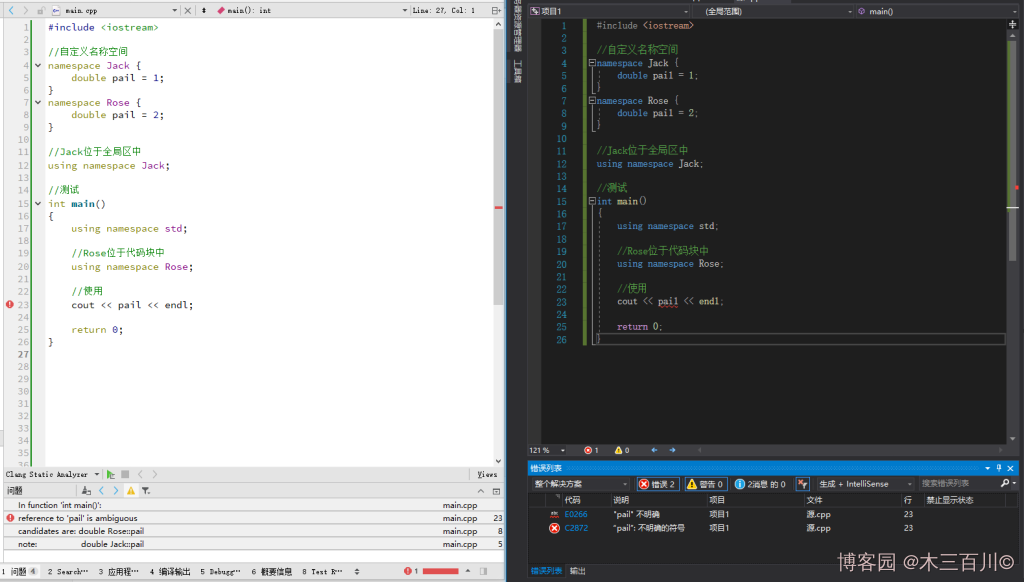## 4 多个同名变量共存

### 4.1 在同名全局变量声明前的全局区中使用

``````#include <iostream>

//自定义名称空间
namespace Jack {
double pail = 1;
}

//在同名全局变量声明前的全局区中使用
using namespace Jack;

//同名全局变量
double pail = 2;

//测试
int main()
{
using namespace std;

//同名局部变量
double pail = 3;

//使用
cout << pail << endl;       //结果为3
cout << ::pail << endl;     //结果为2
cout << Jack::pail << endl; //结果为1

return 0;
}
``````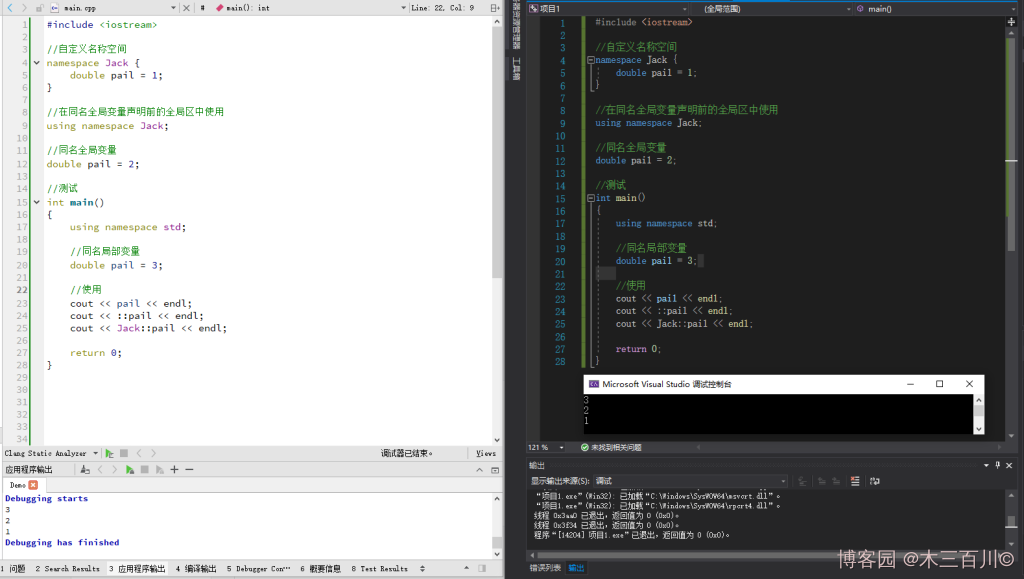### 4.2 在同名全局变量声明后的全局区中使用

``````#include <iostream>

//自定义名称空间
namespace Jack {
double pail = 1;
}

//同名全局变量
double pail = 2;

//在同名全局变量声明后的全局区中使用
using namespace Jack;

//测试
int main()
{
using namespace std;

//同名局部变量
double pail = 3;

//使用
cout << pail << endl;       //结果为3
cout << ::pail << endl;     //结果为2
cout << Jack::pail << endl; //结果为1

return 0;
}
``````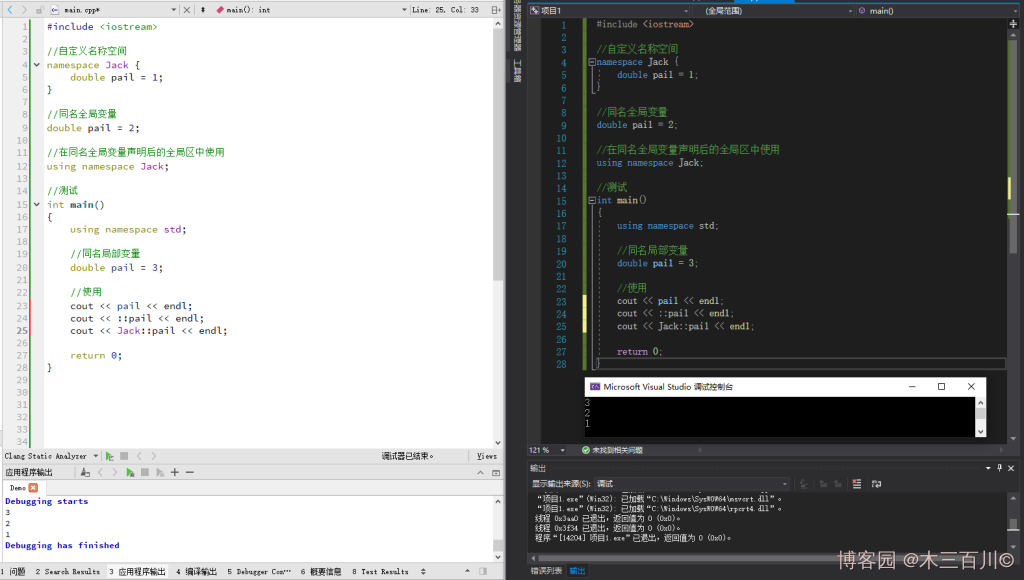### 4.3 在同名局部变量声明前的代码块中使用

``````#include <iostream>

//自定义名称空间
namespace Jack {
double pail = 1;
}

//同名全局变量
double pail = 2;

//测试
int main()
{
using namespace std;

//在同名局部变量声明前的代码块中使用
using namespace Jack;

//同名局部变量
double pail = 3;

//使用
cout << pail << endl;       //结果为3
cout << ::pail << endl;     //结果为2
cout << Jack::pail << endl; //结果为1

return 0;
}
``````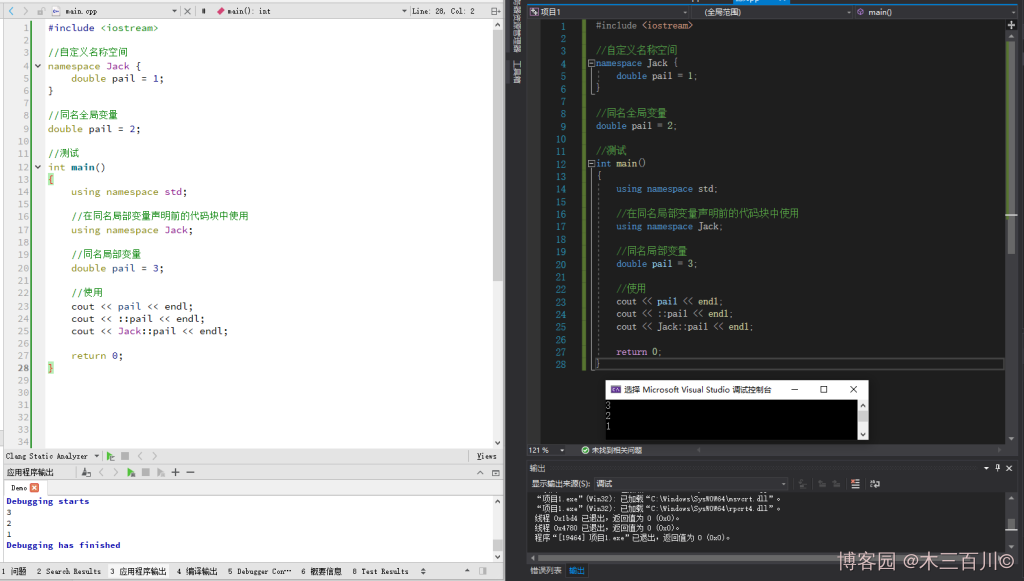### 4.4 在同名局部变量声明后的代码块中使用

``````#include <iostream>

//自定义名称空间
namespace Jack {
double pail = 1;
}

//同名全局变量
double pail = 2;

//测试
int main()
{
using namespace std;

//同名局部变量
double pail = 3;

//在同名局部变量声明后的代码块中使用
using namespace Jack;

//使用
cout << pail << endl;       //结果为3
cout << ::pail << endl;     //结果为2
cout << Jack::pail << endl; //结果为1

return 0;
}
``````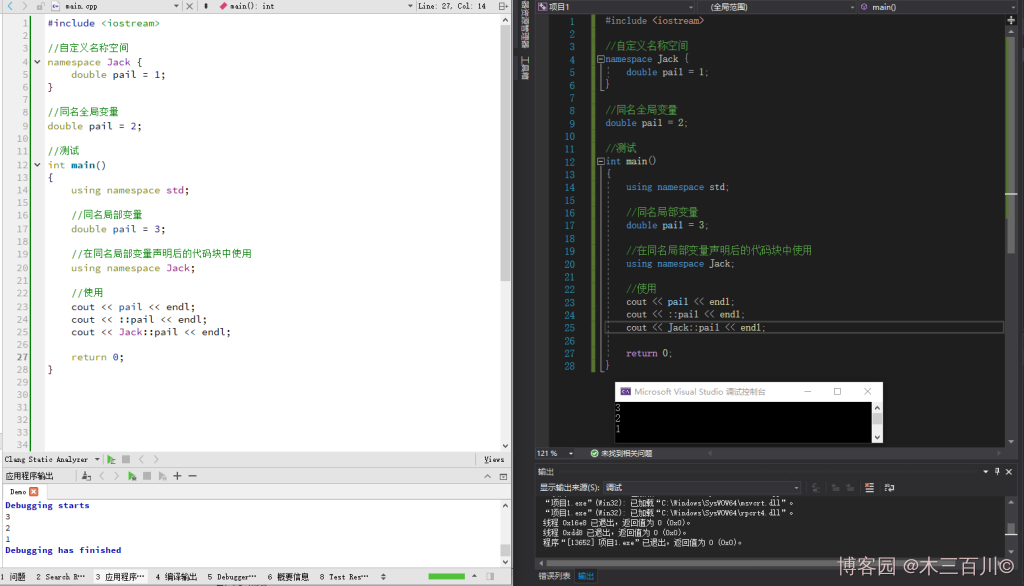## 5 总结

• 若仅存在同名全局变量，不存在同名局部变量，使用 `using` 编译指令后，在作用域的重合区域使用变量时一定会引发名称冲突。
• 若仅存在同名局部变量，不存在同名全局变量，使用 `using` 编译指令将会进行名称解析，不会引发名称冲突，但在代码块中，同名局部变量将隐藏名称空间中的变量。
• 若不同名称空间中存在同名变量，不存在同名全局变量以及同名局部变量，使用 `using` 编译指令后，在作用域的重合区域使用变量时一定会引发名称冲突。
• 若名称空间中的变量、同名全局变量、同名局部局部变量三者同时存在，`using` 编译指令的使用位置不会影响名称解析的结果，且不会引发名称冲突，这正是 `using` 编译指令进行名称解析的效果。

`Jack` 名称空间中的 `pail` 变量为例，将使用 `using` 编译指令时可能遇到的各种情况列表如下，表中的最后一列是指在作用域的重合区域使用变量时是否会引发名称冲突。

1 存在 冲突
2 存在 存在 冲突
3 存在 不冲突
4 存在 存在 不冲突
5 存在 冲突
6 存在 存在 不冲突
7 存在 存在 存在 不冲突
8 不冲突